# Solid geometry, stereometry

Solid geometry is the name for the geometry of three-dimensional Euclidean space.

Stereometry deals with the measurements of volumes of various solid figures (three-dimensional figures) including pyramids, prisms and other polyhedrons; cylinders; cones; truncated cones; and balls bounded by spheres.

1. PrismThe volume of tetrahedral prism is 2.43 m3. Base of prism is a parallelogram in which a side 2,5dm and height ha = 18cm. Calculate the height of the prism.
2. Lamp coneCalculate the surface of a lamp shade shaped of a rotary truncated cone with base diameter 32 cm and 12 cm and height 24 cm.
3. Tetrahedral prism - rhomboid baseCalculate the area and volume tetrahedral prism that has base rhomboid shape and its dimensions are: a = 12 cm, b = 70 mm, v_a = 6 cm, v_h = 1 dm.
4. Cellar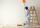Cellar for storing fruit has a rectangular base with sides 14 m and 7 meters. You should paint sidewall to 2 m. How m2 surface must be painted?
5. TentCalculate how many liters of air will fit in the tent that has a shield in the shape of an isosceles right triangle with legs r = 3 m long the height = 1.5 m and a side length d = 5 m.
6. Hexagonal pyramidRegular hexagonal pyramid has dimensions: length edge of the base a = 1.8 dm and the height of the pyramid = 2.4 dm. Calculate the surface area and volume of a pyramid.
7. Pyramid in cubeIn a cube with edge 12 dm long we have inscribed pyramid with the apex at the center of the upper wall of the cube. Calculate the volume and surface area of the pyramid.
8. Cuboid - volume and areasThe cuboid has a volume of 250 cm3, a surface of 250 cm2 and one side 5 cm long. How do I calculate the remaining sides?
9. Bathroom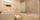How many CZK we pay for lining the perimeter walls of the bathroom with rectangular shape with dimensions 3.5 m and 4 m, high 1.5 m if 1 square m tile cost 300 CZK?
10. GlassHow many glass are needed to produce glass with base regular 5-gon if one base triangle in the base is 4.2 square cm and the height is 10 cm?
11. Area of the cone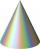Calculate the surface area of the cone, you know the base diameter 25 cm and a height 40 cm.
12. Chimney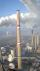Lower circumference of of the chimney is 12.57 m, top circumference is 5.655 m. The slope of the walls is 87°. Determine the height of the chimney.
13. Hexa prismDetermine the volume of hex prism with edge base 4 cm. The body height is 28 cm.
14. Square vs rectangleSquare and rectangle have the same area contents. The length of the rectangle is 9 greater and width 6 less than side of the square. Calculate the side of a square.
15. PrismCalculate the surface area and volume of a prism with a body height h = 10 cm and its base has shape of a rhomboid with sides a = 5.8 cm, b = 3 cm and the distance of its two longer sides is w = 2.4 cm.
16. Surface and volume od cuboidContent area of the square base of cuboid is Sp = 36 cm2 and its height 80 mm. Determine its surface area and volume.
17. Cube from sphere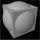What largest surface area (in cm2) can have a cube that was cut out of a sphere with radius 43 cm?
18. Rotating coneCalculate the volume and the surface area of a rotating cone of base radius r = 2.3 dm and a height h = 46 mm.
19. The potDiameter of the pot 38 cm. The height is 30 cm. How many liters of water can fit in the pot?
20. Rectangle poolDetermine dimensions of open pool with a square bottom with a capacity 32 m3 to have painted/bricked walls with least amount of material.

Do you have an interesting mathematical word problem that you can't solve it? Enter it, and we can try to solve it.

To this e-mail address, we will reply solution; solved examples are also published here. Please enter the e-mail correctly and check whether you don't have a full mailbox.

Please do not submit problems from current active competitions such as Mathematical Olympiad, correspondence seminars etc...